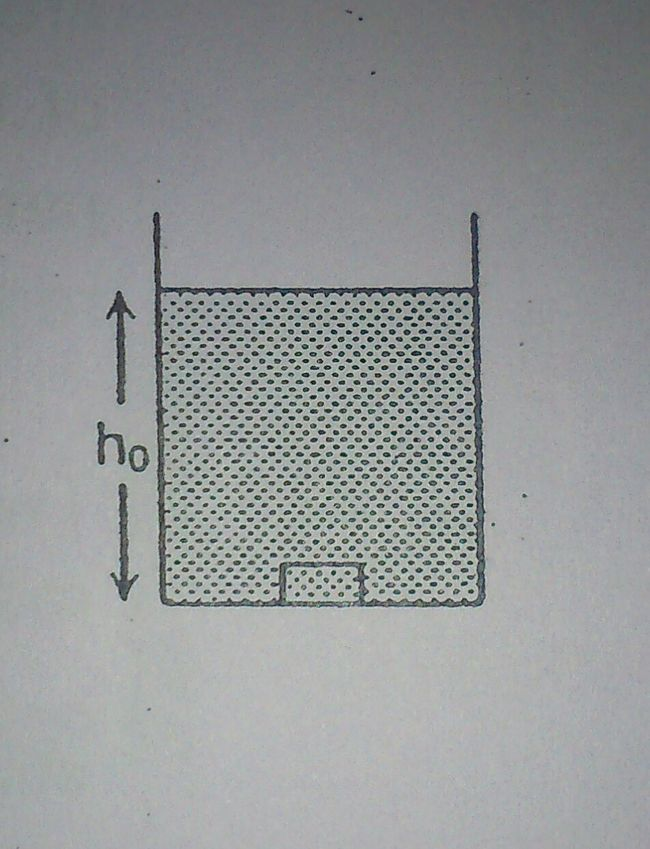# Oscillating block in liquidThe container shown contains a liquid of variable density which varies as $\displaystyle d = d_0 \left( 4 - \dfrac{3y}{h_0} \right) \text{ kg/m}^3$, where $h_0$ is total height of container, $d_0$ is constant and $y$ is measured from the bottom of the container. A solid block whose density is $\dfrac{5}{2} d_0$ and mass 'm' is released from bottom of the container. Given that block will execute SHM and is time period can be written as

$\displaystyle T = 2\pi \sqrt{\dfrac{\alpha h_0}{\beta g}}$

$\text{gcd}(\alpha,\beta) = 1$ and $g$ is acceleration due to gravity. Find $\alpha + \beta$.

Details and Assumptions

• Assume block to be cubical.
×